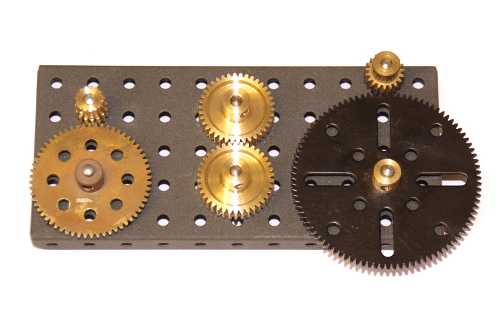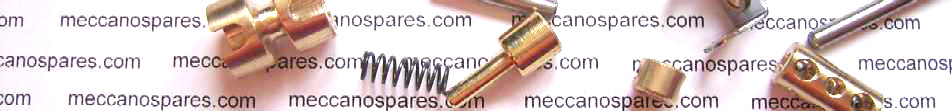Meccano Spares Catalogue

# Meccano Gears - 2

Wednesday, 8 June 2016  |  Admin

## Meccano Gears - 2

### More Simple Gearing.

The larger Meccano kits included a wider range of gears allowing for a wider range of ratios.The holes in the plate are ½" pitch, so you can see the left and centre pair of gears mesh at 1" (25.4mm) centres, the right hand pair of gears mesh at 1½" (38.1mm) centres.

The left hand pair of gears give a 4:1 ratio - the upper pinion (Part 26c) will rotate four times, whilst the lower spur gear (part 27d) will rotate once. This is calculated by 60/15 = 4. In most applications, you need to reduce the rotational speed and increase the torque. If the input is connected to the upper gear, and the output connected to the lower gear the rotational speed will be divided by 4, and the torque roughly multiplied by 4. Additionally, the rotation direction will be reversed.

The centre pair of gears (Part 31) both have the same number of teeth (38), so there is no change in rotational speed or torque, but the rotation direction will be reversed.

These gear pairs (along with the 2:1 and 3:1 mentioned in the previous blog) provide a set of ratios from 1:1 to 4:1 with the same centres, so they can be used for simple gearboxes.

The right hand pair of gears have a larger size difference, but also a wider pitch at 1½". These are the familiar 19 tooth pinion (Part 26) and the large 95 tooth spur gear (Part 27c). These give a ratio of 5:1 (95/19 = 5).

You can, of course, use more than one pair of gears in a gear train, by connecting the output of one pair to the input of the next (by placing both gears on the same axle), you can achieve higher ratios :-

2:1 and 3:1 = 6:1 (2x3=6)

3:1 and 4:1 = 12:1 (3x4=12) or 3:1, 2:1 and 2:1 = 12:1 (3x2x2=12)

5:1 and 2:1 = 10:1 (2x5=10)

 Share TweetMeccano Spares
 View Basket | Wish List | New Account | Sign In
 Search for Meccano Parts: